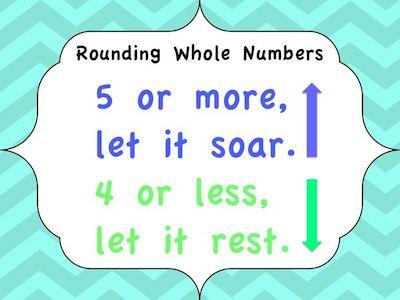# Rounding Numbers – Rules For Fractions

As you probably already know, rounding numbers is a very easy operation that allows you to work with easier numbers. While they have about the same value, they are less exact.

For example, if you want to round the number 341 to the nearest hundred, then you know that the number is 300. And this simply happens because 341 is closer to 300 than to 400.

Make sure to use our free rounding calculator.

And this happens exactly the same way when you want to round off decimals. Let’s say that you want to round off \$1.89 to the nearest dollar, for example. In this case, it would be \$2 since \$1.89 is closer to \$2 than to \$1.

## Rules For Rounding

Before we head onto the rules for fractions, we believe that it makes perfect sense to remember the rules you need to follow when you are rounding numbers:

#1: When the number that you are rounding is followed by 5, 6, 7, 8, or 9, then you need to round the number up. For example, if you want to round the number 38 to the nearest ten, then you will get 40.

#2: When the number that you are rounding is followed by 0, 1, 2, 3, or 4, then you need to round the number down. For example, if you want to round the number 33 to the nearest ten, then you will get 30.

Discover more about rounding to the nearest thousand.

Another aspect that you need to keep in mind when you are rounding numbers is to know what you are rounding to. After all, you can round numbers to the nearest ten, the nearest hundred, the nearest thousand, and so on.

Here are some examples:

Consider the number 4,827.

• 4,827 rounded to the nearest ten is 4,830
• 4,827 rounded to the nearest hundred is 4,800
• 4,827 rounded to the nearest thousand is 5,000

Notice that all the numbers that are at the right of the place that you are rounding, turn into zeros. Here are some more examples:

• 34 rounded to the nearest ten is 30
• 6,809 rounded to the nearest hundred is 6,800
• 1,951 rounded to the nearest thousand is 2,000

Looking to round a number to the nearest hundred?

## Rounding Numbers – Rules For Fractions

The truth is that rounding fractions is also very simple. After all, the rules are exactly the same. The only difference is that instead of rounding to tens, hundreds, thousands, and so on, you round to tenths, hundredths, thousandths, and so on. Here are some examples:

• 7.8199 rounded to the nearest tenth is 7.8
• 1.0621 rounded to the nearest hundredth is 1.06
• 3.8792 rounded to the nearest thousandth is 3.879

Discover how to easily round numbers to the nearest tenth.

One of the things that some students tend to find a bit confusing is when they are rounding with decimals. So, one of the things that can help you in these cases is to only look at the number in the place you are rounding and to the number that follows it.

Here’s an example. Let’s say that you want to round 5.3824791401 to the nearest hundredth. As we just mentioned, you only need to look at the number in the hundredths place – 8 and the number that follows it – 2. So, with this in mind, you can easily round it to 5.38.